# ISEE Upper Level Quantitative : Variables

## Example Questions

### Example Question #11 : Variables

A hat sells for the same pre-tax price in Store A and Store B. Store A then discounts the hat 30%, and Store B discounts it 25%. After the discount, the hat is taxed at a 10% rate in Store A. The hat is taxed at a 5% rate in store B.

Which is greater?

(A) The amount that will be paid for the hat in Store A after discount and tax

(B) The amount that will be paid for the hat in Store B after discount and tax

(B) is greater

It is impossible to determine which is greater from the information given

(A) and (B) are equal

(A) is greater

(B) is greater

Explanation:

Letbe the original price of the hat before discount or tax.

Store A discounts the hat by 30%, meaning that its discounted price before sales tax is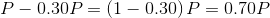The amount paid after sales tax is this price plus 10% of it, or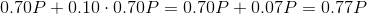Store B discounts the hat by 25%, meaning that its discounted price before sales tax is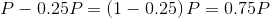The amount paid after sales tax is this price plus 5% of it, or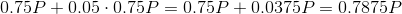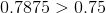andmust be positive, so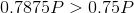.

(B) must be greater regardess of.

### Example Question #11 : How To Multiply Variables

A hat sells for the same price in Store A, where the sales tax is 8%, and Store B, where there is no sales tax. Store A then discounts the hat by 8%; Store B does not discount it.

Which is greater?

(A) The amount that will be paid for the hat in Store A after discount and tax

(B) The amount that will be paid for the hat in Store B

(A) is greater

(B) is greater

(A) and (B) are equal

It is impossible to determine which is greater from the information given

(B) is greater

Explanation:

Letbe the original price of the hat before discount or tax.

Store A discounts the hat by 8%, meaning that its discounted price before sales tax is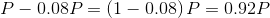.

The amount paid after sales tax is this price plus 8% of it, or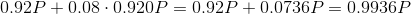.

Since Store B neither discounts the price nor charges sales tax, the amount paid will be the original.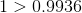, so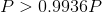.

The hat will cost more in Store B, so (B) is greater.

### Example Question #13 : Variables

Assumeis nonzero. Which of the following is equivalent to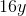?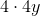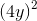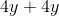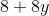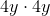Explanation:

Using the associative property of multiplication,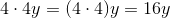.

Using the distributive property,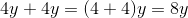.

Using the commutative and associative properties of multiplication,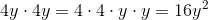and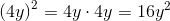.

The expressionis the sum of unlike terms and cannot be simplified further.

The only expression that can be restated asis.

### Example Question #14 : Variablesandare positive integers. Which is the greater quantity?

(A)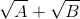(B)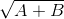(A) and (B) are equal

It is impossible to tell which is greater from the information given

(A) is greater

(B) is greater

(A) is greater

Explanation:

We can compare these positive numbers by comparing their squares; the greater number will have the greater square.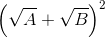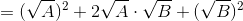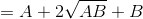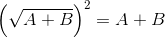Sinceandare positive,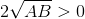and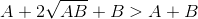.

Therefore,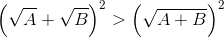and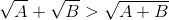.

### Example Question #15 : Variables

Factor: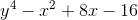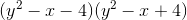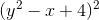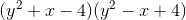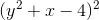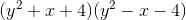Explanation:

We can rewrite as follows: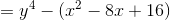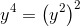, and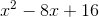is a perfect square polynomial, as seen here: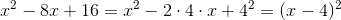so the original polynomial is equal to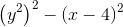This is the difference of squares, so it can be factored as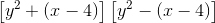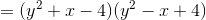### Example Question #16 : Variables

If m is a negative integer, which of the following is an expression that also represents a positive integer?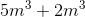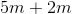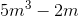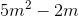Explanation:

The easiest way to solve this problem is to take a negative integer to use for m.

For example,can be used.

Plugging ininto the expression,, we get: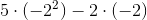This simplifies to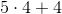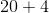Given that 24 is a positive number,is the correct answer.

### Example Question #17 : How To Multiply Variables

When evaluating the expression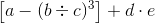,

assuming you know the values of all five variables, what is the last operation that must be performed?

Division

Subtraction

Multiplication

Cubing

Explanation:

By the order of operations, all operations within grouping symbols must be performed first, with the innermost symbols taking precedence. Therefore, the three operations within the brackets - the subtraction, the division, and the cubing - must be performed before the remaining two.

Once these three operations are completed, there remain two more, a division and an addition. Division has precendence in the order of operations, so the last operation performed is the addition.

### Example Question #18 : How To Multiply Variables

Which is the greater quantity?

(a) The number of miles in 100 kilometers

(b) The number of kilometers in 100 miles

Note: You may use the conversion factor 1 mile = 1.609 kilometers.

(b) is the greater quantity

It is impossible to determine which is greater from the information given

(a) is the greater quantity

(a) and (b) are equal

(b) is the greater quantity

Explanation:

Since 1 mile is equivalent to 1.609 kilometers, the number of kilometers equivalent to 100 miles can be found by multiplying 100 by 1.609. Conversely, the number of miles equivalent to 100 kilometers can be found by dividing 100 by 1.609.

You do not have to do the actual math to answer the question. Since the conversion factor is greater than one, multiplying any positive number by this factor yields a result greater than dividing that same number by it. Therefore,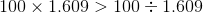,

and the number of kilometers equivalent to 100 miles, (b), is the greater quantity.

### Example Question #19 : How To Multiply Variablesis a positive number. Which is the greater quantity?

(a) The number of inches in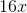feet

(b) The number of ounces in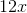pounds

It is impossible to determine which is greater from the information given

(a) is the greater quantity

(b) is the greater quantity

(a) and (b) are equal

(a) and (b) are equal

Explanation:

One foot comprises twelve inches, so multiply the number of feet by conversion factor 12: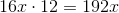inches.

One pound comprises sixteen ounces, so multiply the number of ounces by conversion factor 16: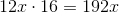ounces.

The two quantities are both equal to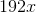.

### Example Question #20 : How To Multiply Variables

Which is the greater quantity?

(a) The number of inches in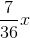yards

(b) The number of days in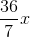weeks

It is impossible to determine which is greater from the information given

(b) is the greater quantity

(a) and (b) are equal

(a) is the greater quantity

(b) is the greater quantity

Explanation:

One yard comprises thirty-six inches, so multiply the number of yards by conversion factor 36: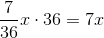One week comprises seven days, so multiply the number of weeks by conversion factor 7: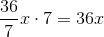(b) is the greater quantity, since, ifis positive,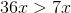.

### All ISEE Upper Level Quantitative Resources